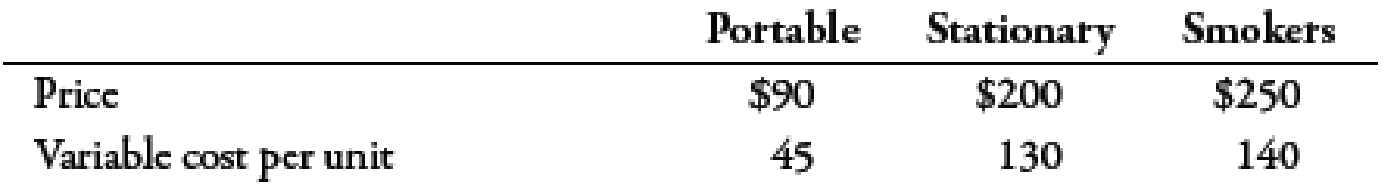Chapter 7, Problem 45EManagerial Accounting: The Corners...

7th Edition
Maryanne M. Mowen + 2 others
ISBN: 9781337115773

Solutions

Chapter
SectionManagerial Accounting: The Corners...

7th Edition
Maryanne M. Mowen + 2 others
ISBN: 9781337115773
Textbook Problem

Texas-Q Company produces and sells barbeque grills. Texas-Q sells three models: a small portable gas grill, a larger stationary gas grill, and the specialty smoker. In the coming year, Texas-Q expects to sell 20,000 portable grills, 50,000 stationary grills, and 5,000 smokers. Information on the three models is as follows:Total fixed cost is \$2,128,500.Required: 1. What is the sales mix of portable grills to stationary grills to smokers? 2. Compute the break-even quantity of each product. 3. Prepare an income statement for Texas-Q for the coming year. What is the overall contribution margin ratio? Use the contribution margin ratio to compute overall breakeven sales revenue. (Note: Round the contribution margin ratio to four decimal places; round the break-even sales revenue to the nearest dollar.) 4. Compute the margin of safety for the coming year.

1.

To determine

Calculate the sales mix of portable grills, stationary grills and smokers.

Explanation

Sales Mix:

Sales mix refers to the proportion of different products that is sold. It is expressed in the ratio of sales of the products. The ratio of sales mix is reduced to the lowest whole units.

Use the following formula to calculate sales mix for portable grills:

Salesmix=NumberofportablegrillsTotalnumberofitems

Substitute 20,000 for number of portable grills and 75,0001 for total number of items in the above formula.

Salesmix=20,00075,000=415

Therefore, sales mix for Portable grills is 415.

Use the following formula to calculate sales mix for stationary grills:

Salesmix=NumberofstationarygrillsTotalnumberofitems

Substitute 50,000 for number of Portable grills and 75,0001 for total number of items in the above formula.

Salesmix=50,00075,000=1015

Therefore, sales mix for stationary grills is 1015

2.

To determine

Calculate the break-even quantity of each product.

3.

To determine

Construct an income statement for Company TQ. Also calculate overall contribution margin ratio and overall break-even sales revenue.

4.

To determine

Calculate margin of safety.

Still sussing out bartleby?

Check out a sample textbook solution.

See a sample solution

The Solution to Your Study Problems

Bartleby provides explanations to thousands of textbook problems written by our experts, many with advanced degrees!

Get Started

Find more solutions based on key concepts